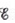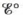# The overall reaction in the lead storage battery is Pb ( s ) + PbO 2 ( s ) + 2H + ( a q ) + 2HSO 4 − ( a q ) → 2PbSO 4 ( s ) + 2H 2 O ( l ) Calculate at 25°C for this battery when [H 2 SO 4 ] = 4.5 M , that is, [H + ] = [HSO 4 − ] = 4.5 M . At 25°C, =2.04 V for the lead storage battery.### Chemistry: An Atoms First Approach

2nd Edition
Steven S. Zumdahl + 1 other
Publisher: Cengage Learning
ISBN: 9781305079243

#### Solutions

Chapter
Section### Chemistry: An Atoms First Approach

2nd Edition
Steven S. Zumdahl + 1 other
Publisher: Cengage Learning
ISBN: 9781305079243
Chapter 17, Problem 71E
Textbook Problem
13 views

## The overall reaction in the lead storage battery is Pb ( s )   +  PbO 2 ( s )   +  2H + ( a q ) +  2HSO 4 − ( a q )       → 2PbSO 4 ( s )   +  2H 2 O ( l ) Calculateat 25°C for this battery when [H2SO4] = 4.5 M, that is, [H+] = [HSO4−] = 4.5 M. At 25°C,=2.04 V for the lead storage battery.

Interpretation Introduction

Interpretation:

The overall reaction taking place in the lead storage battery is given. The value of E at 25°C for this battery at the given concentrations of different ions is to be calculated.

Concept introduction:

The relationship between reduction potential and standard reduction potential value and activities of species present in an electrochemical cell at a given temperature is given by the Nernst equation.

The value of Ecell is calculated using Nernst formula,

E=E°(RTnF)ln(Q)

At room temperature the above equation is specifies as,

E=E°(0.0591n)log(Q)

To determine: The value of E at 25°C for this battery at the given concentrations of different ions.

### Explanation of Solution

Given

The value of concentration of H+ is 0.45M .

The value of concentration of HSO4 is 0.45M .

The value of E°cell is 2.04V .

The reaction taking place in a lead storage battery is given as,

Pb(s)+PbO2(s)+2H+(aq)+2HSO4(aq)2PbSO4(s)+2H2O(l)

The reaction taking place at cathode is,

PbO2(s)+HSO4(aq)+3H+(aq)+2ePbSO4(s)+2H2O(l)

The reaction taking place at anode is,

Pb(s)+HSO4(aq)PbSO4(s)+H+(aq)+2e

The reaction involves the transfer of 2 moles of electrons and the value of E°cell=2

### Still sussing out bartleby?

Check out a sample textbook solution.

See a sample solution

#### The Solution to Your Study Problems

Bartleby provides explanations to thousands of textbook problems written by our experts, many with advanced degrees!

Get Started

Find more solutions based on key concepts
A represents a quantitative observation.

Introductory Chemistry: A Foundation

Vitamin C deficiency symptoms include: red spots. loose teeth. anemia. all of the above.

Nutrition: Concepts and Controversies - Standalone book (MindTap Course List)

Why do you think the half-life of collagen is comparatively long?

Chemistry for Today: General, Organic, and Biochemistry

What impact has recombinant DNA technology had on genetics and society?

Human Heredity: Principles and Issues (MindTap Course List)

Review. For the arrangement shown in Figure P17.40, the inclined plane and the small pulley are frictionless; t...

Physics for Scientists and Engineers, Technology Update (No access codes included)

How is heat different from temperature?

Oceanography: An Invitation To Marine Science, Loose-leaf Versin